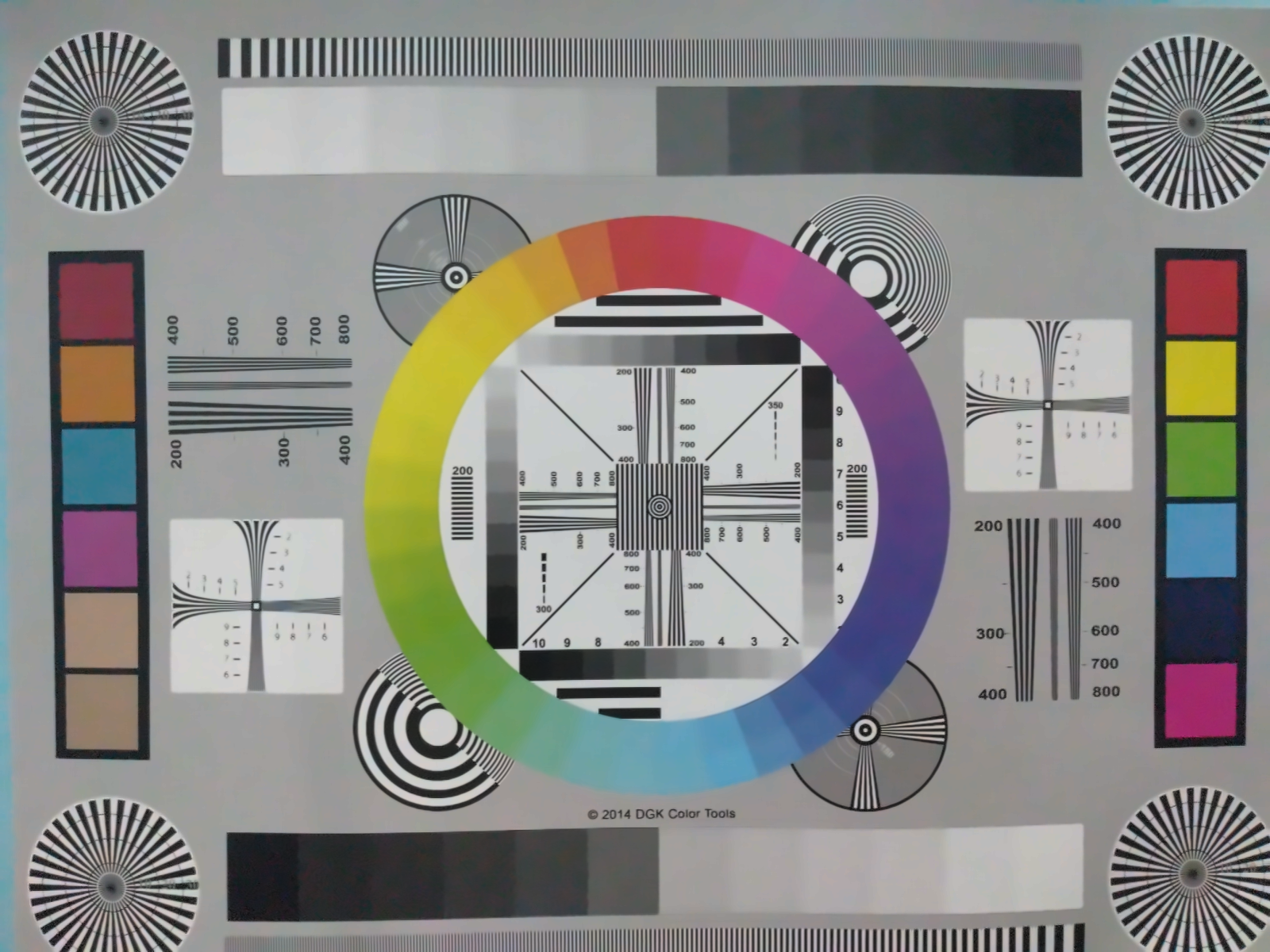• Noise:
• Denoising:

Noise Model

Noise signal that is additive in nature gets added to the original signal to generate a corrupted noisy signal and follows the following rule:

w(x, y) = s(x,y) + n(x,y)

2.2 Multiplicative Noise Model

In this model, noise signal gets multiplied to the original signal. The multiplicative noise model follows the following rule:

w(x, y) = s(x, y) × n(x,y)

Types of noise

• Amplifier Noise: The typical model of amplifier noise is additive, Gaussian, independent at each pixel and independent of the signal intensity. This type of noise has a Gaussian distribution, which has a bell shaped probability distribution function
• Impulsive Noise: Impulsive noise is sometimes called as salt-and- pepper noise or spike noise. This kind of noise is usually seen on images. It represents itself as arbitrarily occurring white and black pixels. An image that contains impulsive noise will have dark pixels in bright regions and bright pixels in dark regions. It can be caused by dead pixels, analog-to-digital converter errors and transmitted bit errors.
• Speckle Noise: Speckle noise is considered as multiplicative noise. It is a granular noise that degrades the quality of images obtained by active image devices such as active radar and synthetic aperture radar (SAR) images. Due to random fluctuations in the return signal from an object in conventional radar that is not big as single image processing element, speckle noise occurs. It increases the mean grey level of a local area. Speckle noise makes image interpretation difficult in SAR images caused mainly due to coherent processing of back-scattered signals from multiple distributed targets.

Algorithms to compare in this series

• Mean filter
• Median filter
• Non-local means
• BM3D